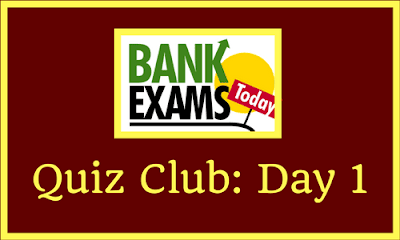# BankExamsToday Quiz Club: Day 1We here have started a new segment called "Quiz Club" We will give you five random questions day that you need to solve and comment below. If is to test your accuracy as well as to give you a quick revision of basic concepts. We will publish the answers next day's quiz. I hope you all would show your enthusiasm for this. Let's start with the easy questions and given below is the BankExamsToday Quiz Club: Day 1

1) A sum of Rs. 5146 is to be divided among x, y and z in such a way that 4 times X’s share, 5 times Y’s share and 7 times Z’s share are all equal. The share of z is?
a) 1259
b) 1345
c) 1240
d) 1230
e) None of these

2) In how many different ways can the letters of the word ‘POLICY’ be arranged so that the vowels are at the two ends?
a) 48
b) 24
c) 720
d) 120
e) None of these

3) The ratio of income of C and D is 6:4 and their expenditure is as 4:2. After a year, each saves Rs. 1800, then the income of D is?
a) 900
b) 3600
c) 5400
d) 1800
e) 2000

4) In an examination, 49% students failed in Science, 26% students failed in mathematics, while 25% failed in both. If the total number of passed students is 650, then how many students did appear in the examination?
a) 650
b) 700
c) 1950
d) 1300
e) None of these

5) The present birth rate is 35 per thousand while the death rate is 14 per thousand. What will be the percentage increase in the rate of the population?
a) 2.1%
b) 2%
c) 3%
d) None of these
e) Cannot be determined

Hurry Up!!!! guys. Do post the solutions in comment section